# Geometric Derivation of Euler-Lagrange Equation

Intuitive geometric derivation.

## Statement

Given a functional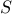: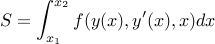Euler-Lagrange says that the function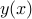at a stationary point of the functionalobeys: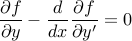Where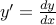.

This result is often proven using integration by parts – but the equation expresses a local condition, and should be derivable using local reasoning.

We will explore an alternate derivation below.

## Motivating Example

Physical systems in stable equilibrium will move to a configuration that locally minimizes their potential energy. For example, consider a chain draped over two pulleys (at height, separated by distance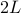), with excess chain resting on the ground.The chain will take a shape between the two pulleys that minimizes its gravitational potential energy. The space is interesting: If the chain is taut, then it will be high above the ground, and have high energy. If the chain is very saggy, it will pull up lots of chain from the ground, and have high energy. In between is the optimal shape.

The potential energy of a given shape of the chainbetween the pulleys is: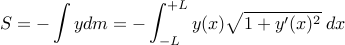We have integrated only along the section between the pulleys, because we can define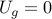at ground level, and ignore constants (the energy of the chain dangling outside the pulleys) and constant factors (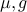).

Now we want to find the functionthat minimizes(subject to the boundary conditions).

## Geometric Derivation

For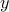to be a stationary point of, small perturbations to the functionmust not alter the value of(to first order). So consider an infinitesimal segment of(grey), discretized around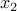. We will consider perturbingatby an amount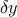(changing from the blue –> red), and examine its effect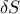on.Recall: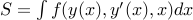Perturbing this point affects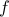in two ways:

1. It alters the value ofat.

2. It alters the derivatives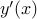around.

The first contribution is simply:The second contribution is in two parts: increasing the derivative on the left, and decreasing the derivative on the right. Based on the figure, we have (before perturbation):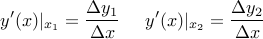And after perturbation:So the change in derivatives is: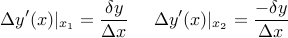This affectsby an amount: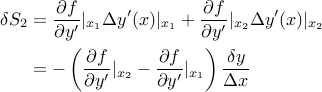Since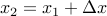, we can write: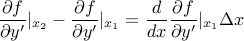So finally, the effect of changing the derivatives is: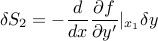And the net effect of perturbing the point is:So clearly, requiringfor small perturbationsanywhere along the function implies the Euler-Lagrange equation:And now both terms have meaning:

1.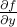: The amount small perturbations affectby directly changing the value.

2.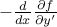: The net amount perturbations affectby changing the derivativesin the neighborhood of. (the derivative to the left increases, and to the right decreases – so the net effect depends on how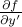changes over the span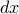).

At a stationary point, these effects must exactly cancel.

For completeness, we derive the solution to the example, and extend it to the case of a fixed-length chain.

### Solution

In this case: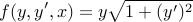Applying Euler-Lagrange directly, we find: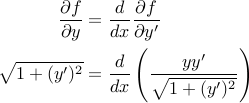We could solve this, but the simpler approach is to use a theorem:

Thm: If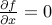, then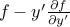is a constant.

Proof:Where the last step is applying the original form of the Euler-Lagrange equation.

Since our particular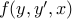is independent of, we can apply this theorem:For constants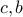chosen to satisfy boundary conditions. This gives us the familiar catenary curve.

### Extension

We know the catenary is also the shape formed if we hang a fixed-length chain between two fixed endpoints. This is no coincidence.

The fixed-length chain problem is a constrained minimization problem, with the same potential energy functional, but the additional constraint that the arclength ofis some specified constant.

Specify constrained problems by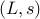– separation distance and chain arclength.

And unconstrained problems by– separation distance and height.

We will show that the solution to this problem is also a catenary, by showing that:

Theorem: For a given constrained-problem, there is a corresponding unconstrained-problemthat contains the constrained-problem as a sub-problem. Therefore the constrained problem must also be a catenary.

Lemma: For any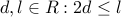, we can always find some unconstrained configurationsuch that the length of chain between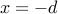andis exactly.

Proof: Refer to the family of unconstrained solutions we found. With centered choice of coordinates,, and the family takes the form:The boundary condition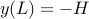determines the parameter. Now, clearly the arclength betweenandis a monotone decreasing function of, with extremes at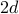and. (eg, for, the arclength turns out to be)

So any value ofin this range is achievable for some– and further, there is somethat gives rise to this(in particular,works).

Result: Now, given a constrained-problem, construct an unconstrained-problem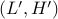such that the length of chain between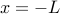and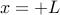is exactly. (which Lemma guarentees we are able to do). Over this span, the unconstrained problem is identical to the constrained problem: arclength, and endpoints separated by. If one situation's optimal-solution (say A) had lower potential energy (over the span) than the other (B), then B could assume the form of A over the span, without violating any constraints but resulting in lower energy. Contradiction of optimality, so both problems must have identical solutions over the span – and the constrained optimal solution is also a catenary.

So we have also derived the solution to the constrained problem. (no Lagrange multipliers necessary!)